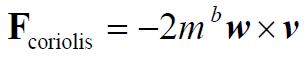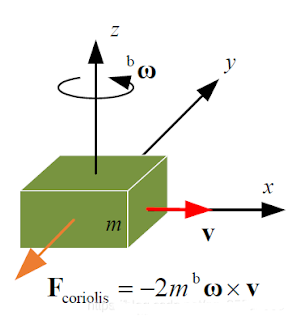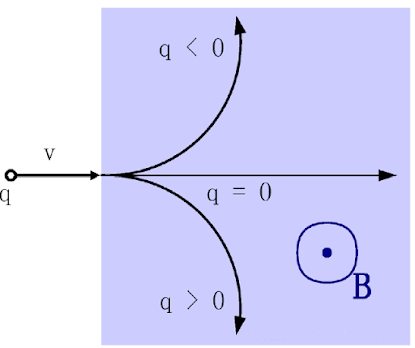Application

# The role of IMU in UAV (MEMS three-axis accelerometer, three-axis gyroscope, three-axis magnetometer)

3-axis accelerometer

(1) Measurement of specific force

A triaxial accelerometer is an inertial sensor that measures the specific force of an object, the overall acceleration without gravity or the non-gravitational force applied per unit mass. When the accelerometer stands still, the accelerometer senses the acceleration of gravity, and the overall acceleration is zero. In free fall, the overall acceleration is the acceleration of gravity, but the internal acceleration of the accelerometer is weightless, and the output of the three-axis accelerometer is zero.(2) Measurement angleThe principle of a triaxial accelerometer can be used to measure angles. Intuitively, as shown in the figure, the amount of spring compression is determined by the angle of the accelerometer to the ground. The specific force can be measured by the compression length of the spring. Therefore, in the absence of external force, the accelerometer can accurately measure the pitch angle and roll angle with no cumulative error.MEMS three-axis accelerometer is based on piezoresistive, piezoelectric and capacitive working principles, and the specific force (pressure or displacement) generated is proportional to the change of resistance, voltage and capacitance respectively. These changes can be acquired by the corresponding amplifier and filter circuits. The disadvantage of the sensor is that it is greatly affected by vibration.

Because of the working principle of its measuring angle, the triaxial accelerometer cannot measure the yaw angle

• Pitch angle and roll angle can be measuredpitching angleThree-axis gyroscope

Function: Used for the calculation of angular velocity and angle after integration of angular velocity in UAV

Principle: To understand the principle of a three-axis gyroscope, the first thing to know is the Coriolis force

Coriolis forces

When a particle moves in a straight line with respect to an inertial frame, because of its inertia, it follows a curve with respect to a rotating frame. Based on the rotation system, we think that there is a force driving the particle trajectory to form a curve. The Coriolis force is a description of this shift, expressed asIn other words, when the motion of a straight line is placed in a rotating system, the trajectory of a straight line will be offset. In fact, the motion of a straight line is not affected by the

force. This virtual force is called the Coriolis forcTherefore, we choose two objects in the gyroscope. They are in constant motion, and the phase difference of their motion is -180 degrees, that is, the two mass blocks move in opposite directions with the same size. They produce opposite Coriolis forces, which force the two corresponding capacitor plates to move, resulting in a capacitance difference change. The change in capacitance is proportional to the angular velocity of rotation. The change of rotation angle can be obtained by the capacitance.

Triaxial magnetometer

The magnetometer provides data on the magnetic field that the device is subjected to along the XYZ axis. This data is then fed into the microcontroller’s algorithm to provide the heading angle associated with the magnetic North Pole. This information can be used to detect geographic position.

The magnetometer uses three mutually perpendicular magnetoresistance sensors, and each axial sensor detects the strength of the geomagnetic field in that direction.An alloy material with a crystalline structure is shown in the figure above. They are very sensitive to the external magnetic field, the strength of the magnetic field will lead to changes in the resistance value of the reluctance sensor.In addition, the triaxial magnetometer can also use the Lorentz force principle, the current flows through the magnetic field to generate force, thus driving changes such as capacitance.